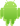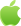# keras.layer.input()用法说明

## 最佳答案：

Keras是一种高级神经网络API，可以运行于TensorFlow、CNTK或Theano之上。Keras.layer.input()是Keras的一种输入层，本文将从多个角度分析这个函数的用法。一、函数介绍

Keras.layer.input()主要用于创建模型的输入层，返回一个张量。函数定义如下：

keras.layers.Input(shape=None, batch_size=None, name=None, dtype=None, sparse=False, tensor=None, **kwargs)

1. shape：输入张量的形状，不包括批量大小。例如，shape=(32,)表示输入的是32维向量，shape=(None, 32)表示输入的是任意尺寸的32维向量。

2. batch_size：可选的固定批量大小。

3. name：层的名称。

4. dtype：输入的数据类型。

5. sparse：布尔值，指定输入是否为稀疏矩阵。

6. tensor：可选的现有张量，用于将该层连接到前面的计算图中。

7. kwargs：其他参数。

1. 创建模型的输入层

Keras.layer.input()主要用于创建模型的输入层，如下所示：

from keras.layers import Input, Dense

inputs = Input(shape=(784,))

x = Dense(64, activation='relu')(inputs)

x = Dense(64, activation='relu')(x)

predictions = Dense(10, activation='softmax')(x)

2. 连接已有模型

Keras.layer.input()还可以用于连接已有模型，如下所示：

from keras.models import Model

from keras.layers import Input, Dense

# 创建一个模型

inputs1 = Input(shape=(784,))

x1 = Dense(64, activation='relu')(inputs1)

x1 = Dense(64, activation='relu')(x1)

predictions1 = Dense(10, activation='softmax')(x1)

model1 = Model(inputs=inputs1, outputs=predictions1)

# 创建另一个模型

inputs2 = Input(shape=(784,))

x2 = Dense(64, activation='relu')(inputs2)

x2 = Dense(64, activation='relu')(x2)

predictions2 = Dense(10, activation='softmax')(x2)

model2 = Model(inputs=inputs2, outputs=predictions2)

# 将两个模型连接起来

merged = keras.layers.concatenate([model1.output, model2.output])

merged = Dense(10, activation='softmax')(merged)

model3 = Model(inputs=[model1.input, model2.input], outputs=merged)

1. 实例描述

- 输入层

- 卷积层（32个卷积核，大小为(3,3)，使用relu激活函数）

- 池化层（使用MaxPooling2D函数，池化大小为(2,2)）

- 卷积层（64个卷积核，大小为(3,3)，使用relu激活函数）

- 池化层（使用MaxPooling2D函数，池化大小为(2,2)）

- 全连接层（使用Dense函数，节点数为128，使用relu激活函数）

- 输出层（使用Dense函数，节点数为10，使用softmax激活函数）

2. 实例代码

from keras.models import Model

from keras.layers import Input, Dense, Conv2D, MaxPooling2D, Flatten

from keras.utils import to_categorical

from keras.datasets import mnist

# 加载数据

(x_train, y_train), (x_test, y_test) = mnist.load_data()

# 数据预处理

x_train = x_train.reshape(-1, 28, 28, 1) / 255.

x_test = x_test.reshape(-1, 28, 28, 1) / 255.

y_train = to_categorical(y_train, 10)

y_test = to_categorical(y_test, 10)

# 创建模型

inputs = Input(shape=(28, 28, 1))

x = Conv2D(32, (3,3), activation='relu', padding='same')(inputs)

x = MaxPooling2D((2,2))(x)

x = Conv2D(64, (3,3), activation='relu', padding='same')(x)

x = MaxPooling2D((2,2))(x)

x = Flatten()(x)

x = Dense(128, activation='relu')(x)

predictions = Dense(10, activation='softmax')(x)

model = Model(inputs=inputs, outputs=predictions)

# 编译模型

# 训练模型

model.fit(x_train, y_train, batch_size=128, epochs=10, validation_data=(x_test, y_test))2022-03-29 更新iPhone下载Android下载